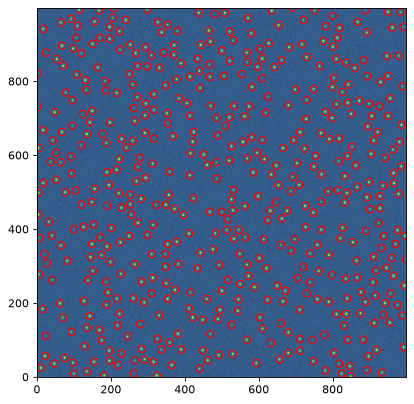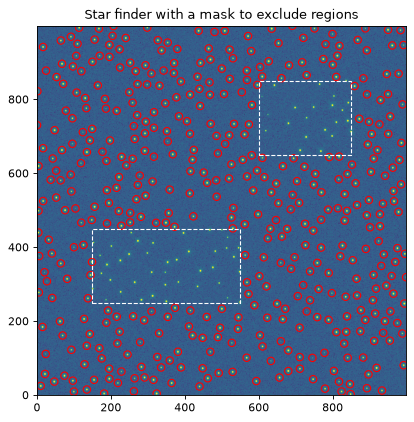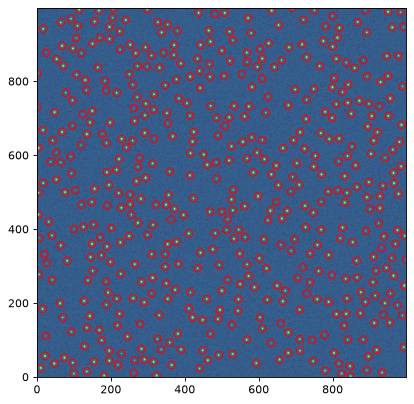# Source Detection (photutils.detection)¶

## Introduction¶

One generally needs to identify astronomical sources in their data before they can perform photometry or morphological measurements. Photutils provides two functions designed specifically to detect point-like (stellar) sources in an astronomical image. Photutils also provides a function to identify local peaks in an image that are above a specified threshold value.

For general-use source detection and extraction of both point-like and extended sources, please see Image Segmentation (photutils.segmentation).

## Detecting Stars¶

Photutils includes two widely-used tools that are used to detect stars in an image, DAOFIND and IRAF’s starfind.

DAOStarFinder is a class that provides an implementation of the DAOFIND algorithm (Stetson 1987, PASP 99, 191). It searches images for local density maxima that have a peak amplitude greater than a specified threshold (the threshold is applied to a convolved image) and have a size and shape similar to a defined 2D Gaussian kernel. DAOStarFinder also provides an estimate of the objects’ roundness and sharpness, whose lower and upper bounds can be specified.

IRAFStarFinder is a class that implements IRAF’s starfind algorithm. It is fundamentally similar to DAOStarFinder, but DAOStarFinder can use an elliptical Gaussian kernel. One other difference in IRAFStarFinder is that it calculates the objects’ centroid, roundness, and sharpness using image moments.

As an example, let’s load an image from the bundled datasets and select a subset of the image. We will estimate the background and background noise using sigma-clipped statistics:

>>> from astropy.stats import sigma_clipped_stats
>>> from photutils import datasets
>>> data = hdu.data[0:401, 0:401]
>>> mean, median, std = sigma_clipped_stats(data, sigma=3.0)
>>> print((mean, median, std))
(3668.09661145823, 3649.0, 204.41388592022315)


Now we will subtract the background and use an instance of DAOStarFinder to find the stars in the image that have FWHMs of around 3 pixels and have peaks approximately 5-sigma above the background. Running this class on the data yields an astropy Table containing the results of the star finder:

>>> from photutils import DAOStarFinder
>>> daofind = DAOStarFinder(fwhm=3.0, threshold=5.*std)
>>> sources = daofind(data - median)
>>> for col in sources.colnames:
...     sources[col].info.format = '%.8g'  # for consistent table output
>>> print(sources)
id xcentroid ycentroid sharpness  ... sky peak    flux       mag
--- --------- --------- ---------- ... --- ---- --------- ------------
1 144.24757 6.3797904 0.58156257 ...   0 6903 5.6976747   -1.8892441
2 208.66907 6.8205805 0.48348966 ...   0 7896 6.7186388   -2.0682032
3 216.92614 6.5775933 0.69359525 ...   0 2195 1.6662764  -0.55436758
4 351.62519 8.5459013 0.48577834 ...   0 6977 5.8970385   -1.9265849
5 377.51991 12.065501 0.52038488 ...   0 1260 1.1178252  -0.12093477
...       ...       ...        ... ... ...  ...       ...          ...
282 267.90091 398.61991 0.27117231 ...   0 9299 5.4379278   -1.8385836
283 271.46959 398.91242 0.36738752 ...   0 8028 5.0693475   -1.7623802
284 299.05003 398.78469 0.25895667 ...   0 9072 5.5584641    -1.862387
285 299.99359 398.76661 0.29412474 ...   0 9253 5.3233471    -1.815462
286 360.44533 399.52381 0.37315624 ...   0 8079 6.9203438   -2.1003192
Length = 286 rows


Let’s plot the image and mark the location of detected sources:

>>> import numpy as np
>>> import matplotlib.pyplot as plt
>>> from astropy.visualization import SqrtStretch
>>> from astropy.visualization.mpl_normalize import ImageNormalize
>>> from photutils import CircularAperture
>>> positions = np.transpose((sources['xcentroid'], sources['ycentroid']))
>>> apertures = CircularAperture(positions, r=4.)
>>> norm = ImageNormalize(stretch=SqrtStretch())
>>> plt.imshow(data, cmap='Greys', origin='lower', norm=norm)
>>> apertures.plot(color='blue', lw=1.5, alpha=0.5)Regions of the input image can be masked by using the mask keyword with the DAOStarFinder or IRAFStarFinder instance. This simple examples uses DAOStarFinder and masks two rectangular regions. No sources will be detected in the masked regions:

>>> from photutils import DAOStarFinder
>>> daofind = DAOStarFinder(fwhm=3.0, threshold=5.*std)
>>> for col in sources.colnames:
>>>     sources[col].info.format = '%.8g'  # for consistent table output
>>> print(sources)## Local Peak Detection¶

Photutils also includes a find_peaks() function to find local peaks in an image that are above a specified threshold value. Peaks are the local maxima above a specified threshold that are separated by a specified minimum number of pixels.

By default, the returned pixel coordinates are always integer-valued (i.e., no centroiding is performed, only the peak pixel is identified). However, a centroiding function can be input via the centroid_func keyword to find_peaks() to compute centroid coordinates with subpixel precision.

find_peaks() supports a number of additional options, including searching for peaks only within a specified footprint. Please see the find_peaks() documentation for more options.

As a simple example, let’s find the local peaks in an image that are 5 sigma above the background and a separated by at least 5 pixels:

>>> from astropy.stats import sigma_clipped_stats
>>> from photutils.datasets import make_100gaussians_image
>>> from photutils import find_peaks
>>> data = make_100gaussians_image()
>>> mean, median, std = sigma_clipped_stats(data, sigma=3.0)
>>> threshold = median + (5. * std)
>>> tbl = find_peaks(data, threshold, box_size=11)
>>> tbl['peak_value'].info.format = '%.8g'  # for consistent table output
>>> print(tbl[:10])  # print only the first 10 peaks
x_peak y_peak peak_value
------ ------ ----------
233      0  27.477852
493      6  20.404769
207     11  24.075798
258     12  17.395025
366     12  18.729726
289     22  35.853276
380     29  19.261986
442     31  30.239994
359     36  19.771626
471     38   25.45583


And let’s plot the location of the detected peaks in the image:

>>> import numpy as np
>>> import matplotlib.pyplot as plt
>>> from astropy.visualization import simple_norm
>>> from astropy.visualization.mpl_normalize import ImageNormalize
>>> from photutils import CircularAperture
>>> positions = np.transpose((tbl['x_peak'], tbl['y_peak']))
>>> apertures = CircularAperture(positions, r=5.)
>>> norm = simple_norm(data, 'sqrt', percent=99.9)
>>> plt.imshow(data, cmap='Greys_r', origin='lower', norm=norm)
>>> apertures.plot(color='#0547f9', lw=1.5)
>>> plt.xlim(0, data.shape-1)
>>> plt.ylim(0, data.shape-1)## Reference/API¶

This subpackage contains tools for detecting sources in an astronomical image.

### Functions¶

 detect_threshold(data, nsigma[, background, …]) Calculate a pixel-wise threshold image that can be used to detect sources. find_peaks(data, threshold[, box_size, …]) Find local peaks in an image that are above above a specified threshold value.

### Classes¶

 DAOStarFinder(threshold, fwhm[, ratio, …]) Detect stars in an image using the DAOFIND (Stetson 1987) algorithm. IRAFStarFinder(threshold, fwhm[, …]) Detect stars in an image using IRAF’s “starfind” algorithm. StarFinderBase Abstract base class for star finders.